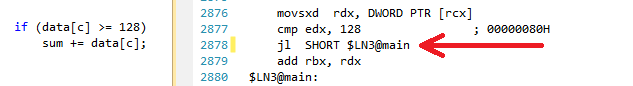数组遍历的性能分析

Posted by Gityuan on August 30, 2015

问题

``````#include <algorithm>
#include <ctime>
#include <iostream>

int main()
{
// 生成数据
const unsigned arraySize = 32768;
int data[arraySize];

for (unsigned c = 0; c < arraySize; ++c)
data[c] = std::rand() % 256;

// !!! 排过序，接下来的循环运行会更快
std::sort(data, data + arraySize);

// 开始计时
clock_t start = clock();
long long sum = 0;

for (unsigned i = 0; i < 100000; ++i)
{
//主循环
for (unsigned c = 0; c < arraySize; ++c)
{
if (data[c] >= 128)
sum += data[c];
}
}

//结束计时
double elapsedTime = static_cast<double>(clock() - start) / CLOCKS_PER_SEC;

std::cout << elapsedTime << std::endl;
std::cout << "sum = " << sum << std::endl;
}
``````
• 对于去掉`std::sort(data, data + arraySize)`，代码运行时间为11.54s.
• 对于已排序的数据，代码运行时间为1.93s.

``````import java.util.Arrays;
import java.util.Random;

public class Main
{
public static void main(String[] args)
{
// 生成数据
int arraySize = 32768;
int data[] = new int[arraySize];

Random rnd = new Random(0);
for (int c = 0; c < arraySize; ++c)
data[c] = rnd.nextInt() % 256;

// !!! 排过序，接下来的循环运行会更快
Arrays.sort(data);

// 开始计时
long start = System.nanoTime();
long sum = 0;

for (int i = 0; i < 100000; ++i)
{
//主循环
for (int c = 0; c < arraySize; ++c)
{
if (data[c] >= 128)
sum += data[c];
}
}

//结束计时
System.out.println((System.nanoTime() - start) / 1000000000.0);
System.out.println("sum = " + sum);
}
}
``````

解答• 如果猜测对了，火车不需要停下来，可以直接继续前行。
• 如果猜测错了，火车需要停下来，回退，然后切换线路。火车再重新进入另一个线路。• 如果猜测对了，继续执行
• 如果猜测错了，需要清空管道，分支回滚，然后重新进入另一个条分支。

``````if (data[c] >= 128)
sum += data[c];
``````

``````T = branch taken
N = branch not taken

data[] = 0, 1, 2, 3, 4, ... 126, 127, 128, 129, 130, ... 250, 251, 252, ...
branch = N  N  N  N  N  ...   N    N    T    T    T  ...   T    T    T  ...

= NNNNNNNNNNNN ... NNNNNNNTTTTTTTTT ... TTTTTTTTTT  (easy to predict)
``````

``````data[] = 226, 185, 125, 158, 198, 144, 217, 79, 202, 118,  14, 150, 177, 182, 133, ...
branch =   T,   T,   N,   T,   T,   T,   T,  N,   T,   N,   N,   T,   T,   T,   N  ...

= TTNTTTTNTNNTTTN ...   (completely random - hard to predict)
``````

``````if (data[c] >= 128)
sum += data[c];
``````

``````int t = (data[c] - 128) >> 31;
sum += ~t & data[c];
``````

``````<< :左移运算符，num << 1，等价于num*2;
>> :右移运算符，num >> 1，等价于num/2; 空位以符号位来补齐
>>> :无符号右移，忽略符号位(最高位)，空位都以0补齐；
~： 非运算符，当位为0，则结果为1；，但位为1，则结果为0；
``````

• 当data[c]大于128时，`int t = (data[c] - 128) >> 31`，根据带符号的右移位运算法则，得到t=0，则~t=-1(即所有的位都为1); 从而`~t & data[c] = data[c]`，故等效sum += data[c];
• 当data[c]小于或者等于128时，`int t = (data[c] - 128) >> 31`，根据带符号的右移位运算法则，得到t=-1，则~t=0; 从而~t & data[c] = 0，故等效此未进入分支;

Benchmarks: Core i7 920 @ 3.5 GHz

C++ - Visual Studio 2010 - x64 Release

``````//  原始-随机的
seconds = 11.777

//  原始-已排序的
seconds = 2.352

//  改进后-随机的
seconds = 2.564

//  改进后-排序的
seconds = 2.587
``````

Java - Netbeans 7.1.1 JDK 7 - x64

``````//  原始-随机的
seconds = 10.93293813

//  原始-已排序的
seconds = 5.643797077

//  改进后-随机的
seconds = 3.113581453

//  改进后-排序的
seconds = 3.186068823
``````

• 原始方法: 有非常大的差异在排序与未排序的数据之间
• 改进后方法： 排序与未排序的数据基本无差异
• C++语言下，改进后的方法，对于排序过的数据计算稍微比原始的方法慢一些。

• 在x64上 GCC 4.6.1（-O3 或者 -ftree-vectorize）的环境下，是可以生成条件移动(conditional move，没有想到合适的译文，暂时称为条件移动)，因为在排序与未排序的数据没有区别，一样快。
• VC++ 2010是无法为分支生成条件移动。
• Intel Compiler 11做了一些特殊处理。它交换了两个循环，从而把不可预测的分支提到外循环。因此，它不仅是避免了预测失误，也是快两倍于VC++和GCC。换句话说，ICC通过测试循环来获取更好的性能…
• 如果你给Intel编译器的改进代码，它只是向右向量化…跟含有循环交换的分支一样快。